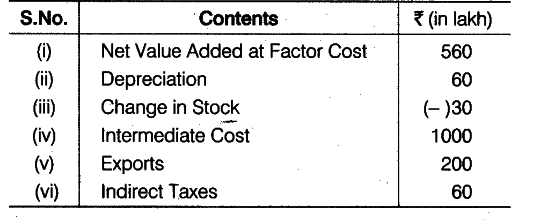# Calculate the sales from the following data

Calculate sales from the following data:Gross Value Added at Market Price (\$GV{{A}{MP}}\$)
= \$NV{{A}
{FC}}\$ + Net Indirect Taxes + Depreciation = 560 + 60 + 60 = Rs 680 lakh
\$GV{{A}_{MP}}\$ = Value of Output - Intermediate Cost 680 = Sales + (- 30) - 1000 Sales = 680 + 1000 + 30 = Rs 1710 lakh